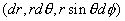The Laplacians,Curls, Divergences, Gradients, line, surface, and volume Elements:
The Laplacians: Here we attempt to give you the Laplacians in various coordinate systems.

Cartesian coordinates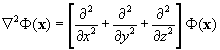Cylindrical coordinates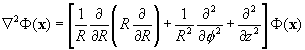Spherical coordinates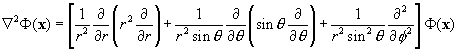The Curls: Here we attempt to give you the Curls in various coordinate systems.

Cartesian coordinates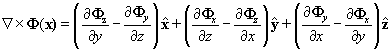Cylindrical coordinates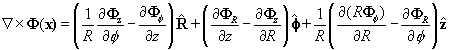Spherical coordinates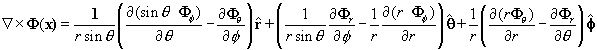The Divergences: Here we attempt to give you the Divergences in various coordinate systems.

Cartesian coordinates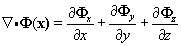Cylindrical coordinates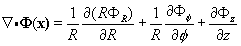Spherical coordinates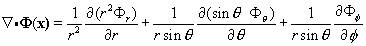The Gradients : Here we attempt to give you the Gradient in various coordinate systems.

Cartesian coordinates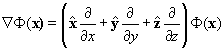Cylindrical coordinates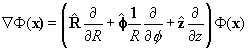Spherical coordinates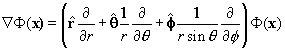The Elements: Here we attempt to give you the line, area, and volume elements in various coordinate systems.

Cartesian coordinates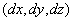Cylindrical coordinates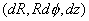Spherical coordinates